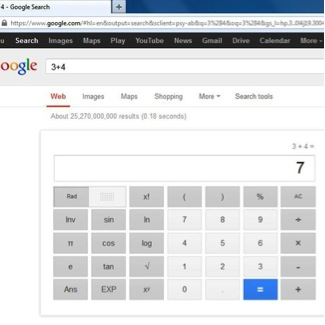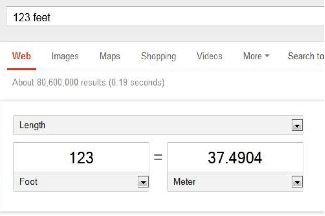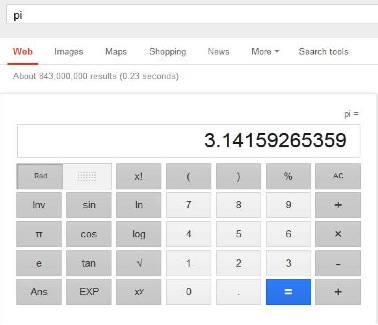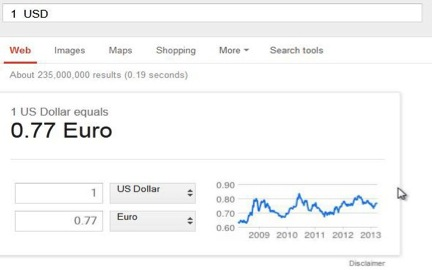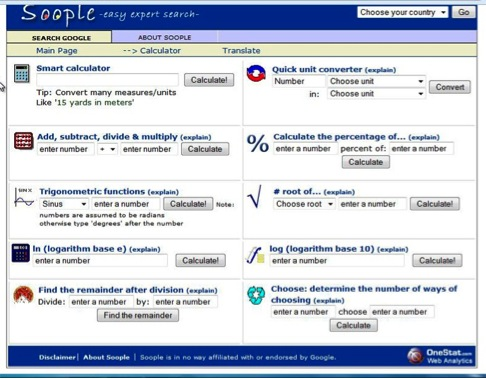## How to Use Google Calculator

In this tutorial you will learn how use Google calculator to perform basic and advanced arithmetical functions. You will also be shown how to convert measure units, find values of constants and convert world currencies with Google calculator. At the end of the tutorial, we will show you how to access a graphical interface for all the basic functions.

Step 1:

You can now solve mathematical problems with Google’s built-in calculator function.
Simply enter the expression you’d like evaluated in Google’s web search box and click ENTER or click the Google Search button.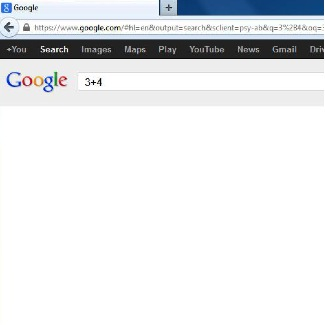Step 2: A full set of basic mathematical operations: addition, substraction, multiplication and division, as well as advanced functions can be easily performed this way.